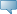## Detailed Course Information

Winter 2021
Oct 16, 2021Select the desired Level or Schedule Type to find available classes for the course.

 MATH 2080 - Introduction to Analysis (Lab required) The course is intended for students in mathematically rich disciplines. Fundamental properties of the real number system as a complete ordered field, Archimedean property, existence of square roots, density of rational numbers, uncountability of real numbers. Sequences, subsequences, limit theorems, monotonicity, Bolzano-Weierstrass theorem, Cauchy sequences. Rigorous treatment of limits and continuity of functions of one and several variables. Uniform continuity. Applications. May not be held with MATH 2081 or the former MATH 2202. Prerequisites: [MATH 1232 (C) or MATH 1690 (C) or MATH 1700 (B) or MATH 1701 (B) or MATH 1710 (B)] and [MATH 1220 (C) or MATH 1300 (B) or MATH 1301 (B)] and [MATH 1240 (C) or MATH 1241 (C)]. - 3.0 Credit hours Science, Science lab Mathematics Department Course Attributes: Mathematics Requirement, Science Requirement for BA, Science Restrictions: Must be enrolled in one of the following Levels:            Undergraduate Must be enrolled in one of the following Campuses:            Main (Fort Garry & Bannatyne)